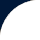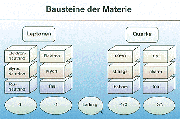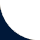Contact:# The OPERA Project

## Physics motivation

The mass of the neutrino is one of the most intriguing questions of particle physics. On the one hand, there is no fundamental principle for the neutrino to be massless; on the other hand, a neutrino mass implies an extension of the Standard Model (Fig.1) of elementary physics. "An answer" would represent a fundamental milestone in particle physics, astrophysics and cosmology. Most likely, the neutrino mass is too small and therefore out of reach of precision studies of the kinematics of decays involving neutrinos. The only possibility to get information is the observation of a process which can only occur if the neutrino has non vanishing mass. Neutrino oscillations are such a process. For a massive neutrino, a given weak interaction eigenstate (nu_e, nu_mu, nu_tau) may be seen as a different eigenstate at some distance from the source. The corresponding probability has an "oscillatory" behavior (hence oscillation) with parameters which can be determined by experiments. In the simplified scheme in which oscillation "occurs" dominantly between a pair of neutrino flavours, they are described by two quantities: the mixing parameter sin²2(Theta) (related to the oscillation amplitude) and the mass squared difference Delta m² between the two mass eigenstates (related to the "frequency oscillation"). The sensitivity of the experimental searches to the above parameters depends on the neutrino energy E and on the distance L of the detector from the neutrino source. The oscillation probability is given by the following equation:The strongest evidence for the existence of neutrino oscillations comes from recent results on the so-called atmospheric neutrino anomaly. The Kamiokande collaboration suggested neutrino oscillations as an explanation of the anomalous ratio of atmospheric muon neutrinos to electron neutrinos and of its zenith angle dependence. The best fit to their data, in terms of nu_mu <-> nu_tau oscillations, gives Delta m² = 1.6x10-2 eV² and sin²2(Theta)=1. The Super-Kamiokande experiment has confirmed the Kamiokande results with a statistics several times larger, making a strong claim for the observation of neutrino oscillations (Fig. 2). A global fit to the Super-Kamiokande data yields m² = 3.2x10-3 eV² and sin²2(Theta)=1 (90% CL). The best fit of Super-Kamiokande and K2K gives the following result: m² = 2.6x10-3. Furthermore the experiments Soudan2, MACRO and SNO yield supporting evidence for the neutrino oscillation.

So far only the disappearance of neutrinos has been experimentaly observed. The conclusive test of the nu_mu <-> nu_tau oscillation hypothesis will be the direct observation of nu_tau appearance in an initially pure nu_mu beam, as proposed in OPERA. The sensitivity of OPERA covers the Delta m² region allowed by the present atmospheric data (Fig. 1). In the case of a positive signal, the observation of even a few nu_tau events will be significant, because of the very low expected background. The number of observed nu_tau events will provide a measurement of the product of the two oscillation parameters sin²2(Theta) and Delta m² in the two flavour mixing scheme. Given the already known constraint of nearly maximum mixing (sin²2(Theta) ~1), OPERA can improve the determination of Delta m².

read more:

read more:

## The OPERA detector> Fig. 1: Standard Modell (© DESY)Fig. 2: OPERA sensitivity, 90% CL